# Pipeline#

class imblearn.pipeline.Pipeline(steps, *, memory=None, verbose=False)[source]#

Pipeline of transforms and resamples with a final estimator.

Sequentially apply a list of transforms, sampling, and a final estimator. Intermediate steps of the pipeline must be transformers or resamplers, that is, they must implement fit, transform and sample methods. The samplers are only applied during fit. The final estimator only needs to implement fit. The transformers and samplers in the pipeline can be cached using `memory` argument.

The purpose of the pipeline is to assemble several steps that can be cross-validated together while setting different parameters. For this, it enables setting parameters of the various steps using their names and the parameter name separated by a ‘__’, as in the example below. A step’s estimator may be replaced entirely by setting the parameter with its name to another estimator, or a transformer removed by setting it to ‘passthrough’ or `None`.

Parameters
stepslist

List of (name, transform) tuples (implementing fit/transform/fit_resample) that are chained, in the order in which they are chained, with the last object an estimator.

memoryInstance of joblib.Memory or str, default=None

Used to cache the fitted transformers of the pipeline. By default, no caching is performed. If a string is given, it is the path to the caching directory. Enabling caching triggers a clone of the transformers before fitting. Therefore, the transformer instance given to the pipeline cannot be inspected directly. Use the attribute `named_steps` or `steps` to inspect estimators within the pipeline. Caching the transformers is advantageous when fitting is time consuming.

verbosebool, default=False

If True, the time elapsed while fitting each step will be printed as it is completed.

Attributes
`named_steps``Bunch`

Access the steps by name.

`classes_`ndarray of shape (n_classes,)

The classes labels.

`n_features_in_`int

Number of features seen during first step `fit` method.

`make_pipeline`

Helper function to make pipeline.

Notes

Warning

A surprising behaviour of the `imbalanced-learn` pipeline is that it breaks the `scikit-learn` contract where one expects `estimmator.fit_transform(X, y)` to be equivalent to `estimator.fit(X, y).transform(X)`.

The semantic of `fit_resample` is to be applied only during the fit stage. Therefore, resampling will happen when calling `fit_transform` while it will only happen on the `fit` stage when calling `fit` and `transform` separately. Practically, `fit_transform` will lead to a resampled dataset while `fit` and `transform` will not.

Examples

```>>> from collections import Counter
>>> from sklearn.datasets import make_classification
>>> from sklearn.model_selection import train_test_split as tts
>>> from sklearn.decomposition import PCA
>>> from sklearn.neighbors import KNeighborsClassifier as KNN
>>> from sklearn.metrics import classification_report
>>> from imblearn.over_sampling import SMOTE
>>> from imblearn.pipeline import Pipeline
>>> X, y = make_classification(n_classes=2, class_sep=2,
... weights=[0.1, 0.9], n_informative=3, n_redundant=1, flip_y=0,
... n_features=20, n_clusters_per_class=1, n_samples=1000, random_state=10)
>>> print(f'Original dataset shape {Counter(y)}')
Original dataset shape Counter({1: 900, 0: 100})
>>> pca = PCA()
>>> smt = SMOTE(random_state=42)
>>> knn = KNN()
>>> pipeline = Pipeline([('smt', smt), ('pca', pca), ('knn', knn)])
>>> X_train, X_test, y_train, y_test = tts(X, y, random_state=42)
>>> pipeline.fit(X_train, y_train)
Pipeline(...)
>>> y_hat = pipeline.predict(X_test)
>>> print(classification_report(y_test, y_hat))
precision    recall  f1-score   support

0       0.87      1.00      0.93        26
1       1.00      0.98      0.99       224

accuracy                           0.98       250
macro avg       0.93      0.99      0.96       250
weighted avg       0.99      0.98      0.98       250
```

Methods

 Transform the data, and apply `decision_function` with the final estimator. `fit`(X[, y]) Fit the model. `fit_predict`(X[, y]) Apply `fit_predict` of last step in pipeline after transforms. `fit_resample`(X[, y]) Fit the model and sample with the final estimator. `fit_transform`(X[, y]) Fit the model and transform with the final estimator. `get_feature_names_out`([input_features]) Get output feature names for transformation. `get_params`([deep]) Get parameters for this estimator. Apply `inverse_transform` for each step in a reverse order. `predict`(X, **predict_params) Transform the data, and apply `predict` with the final estimator. `predict_log_proba`(X, **predict_log_proba_params) Transform the data, and apply `predict_log_proba` with the final estimator. `predict_proba`(X, **predict_proba_params) Transform the data, and apply `predict_proba` with the final estimator. `score`(X[, y, sample_weight]) Transform the data, and apply `score` with the final estimator. Transform the data, and apply `score_samples` with the final estimator. `set_output`(*[, transform]) Set the output container when `"transform"` and `"fit_transform"` are called. `set_params`(**kwargs) Set the parameters of this estimator. Transform the data, and apply `transform` with the final estimator.
property classes_#

The classes labels. Only exist if the last step is a classifier.

decision_function(X)[source]#

Transform the data, and apply `decision_function` with the final estimator.

Call `transform` of each transformer in the pipeline. The transformed data are finally passed to the final estimator that calls `decision_function` method. Only valid if the final estimator implements `decision_function`.

Parameters
Xiterable

Data to predict on. Must fulfill input requirements of first step of the pipeline.

Returns
y_scorendarray of shape (n_samples, n_classes)

Result of calling `decision_function` on the final estimator.

property feature_names_in_#

Names of features seen during first step `fit` method.

fit(X, y=None, **fit_params)[source]#

Fit the model.

Fit all the transforms/samplers one after the other and transform/sample the data, then fit the transformed/sampled data using the final estimator.

Parameters
Xiterable

Training data. Must fulfill input requirements of first step of the pipeline.

yiterable, default=None

Training targets. Must fulfill label requirements for all steps of the pipeline.

**fit_paramsdict of str -> object

Parameters passed to the `fit` method of each step, where each parameter name is prefixed such that parameter `p` for step `s` has key `s__p`.

Returns
selfPipeline

This estimator.

fit_predict(X, y=None, **fit_params)[source]#

Apply `fit_predict` of last step in pipeline after transforms.

Applies fit_transforms of a pipeline to the data, followed by the fit_predict method of the final estimator in the pipeline. Valid only if the final estimator implements fit_predict.

Parameters
Xiterable

Training data. Must fulfill input requirements of first step of the pipeline.

yiterable, default=None

Training targets. Must fulfill label requirements for all steps of the pipeline.

**fit_paramsdict of string -> object

Parameters passed to the `fit` method of each step, where each parameter name is prefixed such that parameter `p` for step `s` has key `s__p`.

Returns
y_predndarray of shape (n_samples,)

The predicted target.

fit_resample(X, y=None, **fit_params)[source]#

Fit the model and sample with the final estimator.

Fits all the transformers/samplers one after the other and transform/sample the data, then uses fit_resample on transformed data with the final estimator.

Parameters
Xiterable

Training data. Must fulfill input requirements of first step of the pipeline.

yiterable, default=None

Training targets. Must fulfill label requirements for all steps of the pipeline.

**fit_paramsdict of string -> object

Parameters passed to the `fit` method of each step, where each parameter name is prefixed such that parameter `p` for step `s` has key `s__p`.

Returns
Xtarray-like of shape (n_samples, n_transformed_features)

Transformed samples.

ytarray-like of shape (n_samples, n_transformed_features)

Transformed target.

fit_transform(X, y=None, **fit_params)[source]#

Fit the model and transform with the final estimator.

Fits all the transformers/samplers one after the other and transform/sample the data, then uses fit_transform on transformed data with the final estimator.

Parameters
Xiterable

Training data. Must fulfill input requirements of first step of the pipeline.

yiterable, default=None

Training targets. Must fulfill label requirements for all steps of the pipeline.

**fit_paramsdict of string -> object

Parameters passed to the `fit` method of each step, where each parameter name is prefixed such that parameter `p` for step `s` has key `s__p`.

Returns
Xtarray-like of shape (n_samples, n_transformed_features)

Transformed samples.

get_feature_names_out(input_features=None)[source]#

Get output feature names for transformation.

Transform input features using the pipeline.

Parameters
input_featuresarray-like of str or None, default=None

Input features.

Returns
feature_names_outndarray of str objects

Transformed feature names.

get_params(deep=True)[source]#

Get parameters for this estimator.

Returns the parameters given in the constructor as well as the estimators contained within the `steps` of the `Pipeline`.

Parameters
deepbool, default=True

If True, will return the parameters for this estimator and contained subobjects that are estimators.

Returns
paramsmapping of string to any

Parameter names mapped to their values.

inverse_transform(Xt)[source]#

Apply `inverse_transform` for each step in a reverse order.

All estimators in the pipeline must support `inverse_transform`.

Parameters
Xtarray-like of shape (n_samples, n_transformed_features)

Data samples, where `n_samples` is the number of samples and `n_features` is the number of features. Must fulfill input requirements of last step of pipeline’s `inverse_transform` method.

Returns
Xtndarray of shape (n_samples, n_features)

Inverse transformed data, that is, data in the original feature space.

property n_features_in_#

Number of features seen during first step `fit` method.

property named_steps#

Access the steps by name.

Read-only attribute to access any step by given name. Keys are steps names and values are the steps objects.

predict(X, **predict_params)[source]#

Transform the data, and apply `predict` with the final estimator.

Call `transform` of each transformer in the pipeline. The transformed data are finally passed to the final estimator that calls `predict` method. Only valid if the final estimator implements `predict`.

Parameters
Xiterable

Data to predict on. Must fulfill input requirements of first step of the pipeline.

**predict_paramsdict of string -> object

Parameters to the `predict` called at the end of all transformations in the pipeline. Note that while this may be used to return uncertainties from some models with return_std or return_cov, uncertainties that are generated by the transformations in the pipeline are not propagated to the final estimator.

New in version 0.20.

Returns
y_predndarray

Result of calling `predict` on the final estimator.

predict_log_proba(X, **predict_log_proba_params)[source]#

Transform the data, and apply `predict_log_proba` with the final estimator.

Call `transform` of each transformer in the pipeline. The transformed data are finally passed to the final estimator that calls `predict_log_proba` method. Only valid if the final estimator implements `predict_log_proba`.

Parameters
Xiterable

Data to predict on. Must fulfill input requirements of first step of the pipeline.

**predict_log_proba_paramsdict of string -> object

Parameters to the `predict_log_proba` called at the end of all transformations in the pipeline.

Returns
y_log_probandarray of shape (n_samples, n_classes)

Result of calling `predict_log_proba` on the final estimator.

predict_proba(X, **predict_proba_params)[source]#

Transform the data, and apply `predict_proba` with the final estimator.

Call `transform` of each transformer in the pipeline. The transformed data are finally passed to the final estimator that calls `predict_proba` method. Only valid if the final estimator implements `predict_proba`.

Parameters
Xiterable

Data to predict on. Must fulfill input requirements of first step of the pipeline.

**predict_proba_paramsdict of string -> object

Parameters to the `predict_proba` called at the end of all transformations in the pipeline.

Returns
y_probandarray of shape (n_samples, n_classes)

Result of calling `predict_proba` on the final estimator.

score(X, y=None, sample_weight=None)[source]#

Transform the data, and apply `score` with the final estimator.

Call `transform` of each transformer in the pipeline. The transformed data are finally passed to the final estimator that calls `score` method. Only valid if the final estimator implements `score`.

Parameters
Xiterable

Data to predict on. Must fulfill input requirements of first step of the pipeline.

yiterable, default=None

Targets used for scoring. Must fulfill label requirements for all steps of the pipeline.

sample_weightarray-like, default=None

If not None, this argument is passed as `sample_weight` keyword argument to the `score` method of the final estimator.

Returns
scorefloat

Result of calling `score` on the final estimator.

score_samples(X)[source]#

Transform the data, and apply `score_samples` with the final estimator.

Call `transform` of each transformer in the pipeline. The transformed data are finally passed to the final estimator that calls `score_samples` method. Only valid if the final estimator implements `score_samples`.

Parameters
Xiterable

Data to predict on. Must fulfill input requirements of first step of the pipeline.

Returns
y_scorendarray of shape (n_samples,)

Result of calling `score_samples` on the final estimator.

set_output(*, transform=None)[source]#

Set the output container when `"transform"` and `"fit_transform"` are called.

Calling `set_output` will set the output of all estimators in `steps`.

Parameters
transform{“default”, “pandas”}, default=None

Configure output of `transform` and `fit_transform`.

• `"default"`: Default output format of a transformer

• `"pandas"`: DataFrame output

• `None`: Transform configuration is unchanged

Returns
selfestimator instance

Estimator instance.

set_params(**kwargs)[source]#

Set the parameters of this estimator.

Valid parameter keys can be listed with `get_params()`. Note that you can directly set the parameters of the estimators contained in `steps`.

Parameters
**kwargsdict

Parameters of this estimator or parameters of estimators contained in `steps`. Parameters of the steps may be set using its name and the parameter name separated by a ‘__’.

Returns
selfobject

Pipeline class instance.

transform(X)[source]#

Transform the data, and apply `transform` with the final estimator.

Call `transform` of each transformer in the pipeline. The transformed data are finally passed to the final estimator that calls `transform` method. Only valid if the final estimator implements `transform`.

This also works where final estimator is `None` in which case all prior transformations are applied.

Parameters
Xiterable

Data to transform. Must fulfill input requirements of first step of the pipeline.

Returns
Xtndarray of shape (n_samples, n_transformed_features)

Transformed data.

## Examples using `imblearn.pipeline.Pipeline`#Multiclass classification with under-sampling

Multiclass classification with under-samplingExample of topic classification in text documents

Example of topic classification in text documents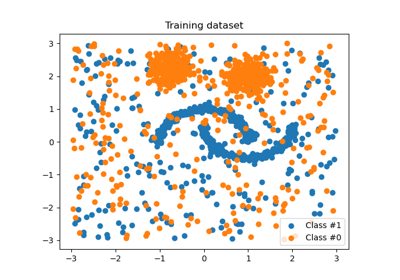Customized sampler to implement an outlier rejections estimator

Customized sampler to implement an outlier rejections estimator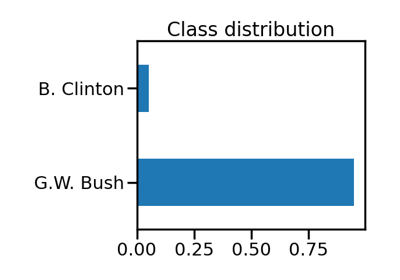Benchmark over-sampling methods in a face recognition task

Benchmark over-sampling methods in a face recognition taskFitting model on imbalanced datasets and how to fight bias

Fitting model on imbalanced datasets and how to fight bias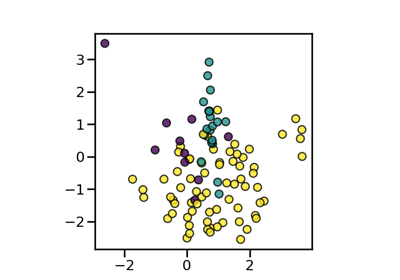Compare sampler combining over- and under-sampling

Compare sampler combining over- and under-samplingBagging classifiers using sampler

Bagging classifiers using samplerEvaluate classification by compiling a report

Evaluate classification by compiling a reportMetrics specific to imbalanced learning

Metrics specific to imbalanced learning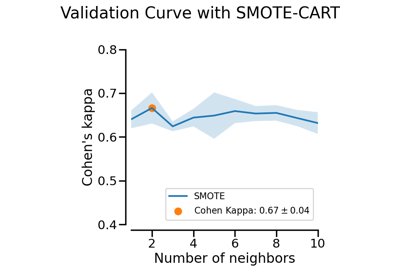Plotting Validation Curves

Plotting Validation Curves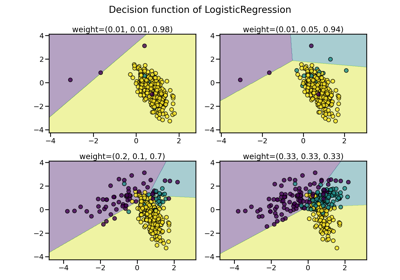Compare over-sampling samplers

Compare over-sampling samplersUsage of pipeline embedding samplers

Usage of pipeline embedding samplers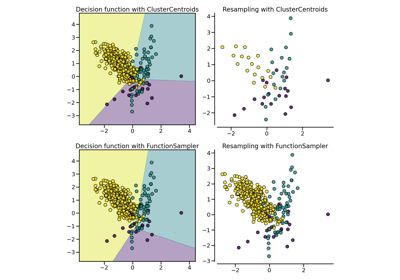Compare under-sampling samplers

Compare under-sampling samplers